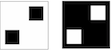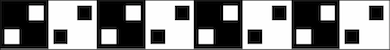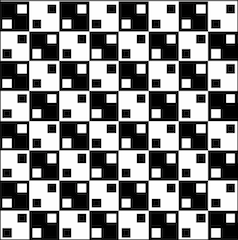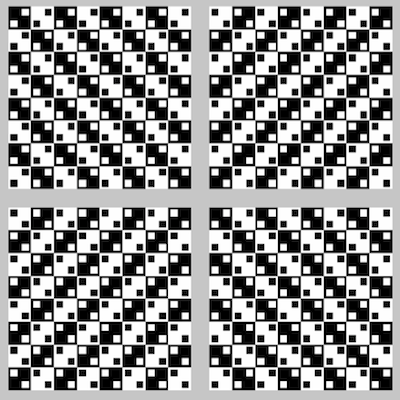# Step Carefully in Math

It's been less than two weeks since the ballots were cast and the electoral votes determined in the presidential election. With seemingly uncannily successful predictions from Nate Silver and Josh Putnam, considerable post-election analysis has focused on the role of mathematics in political science and polling. One should keep in mind, in all such discussions, that keeping an objective, precise view of mathematical content can be difficult. Mathematicians often step carefully and even warily from one logical statement to the next. One misstep can lead to inaccuracy. For example, what fallacy leads to the following claim that 0 = 1?

 (n+1)2 = n2+2n+1 (n+1)2-(2n+1) = n2 (n+1)2-(2n+1)-n(2n+1) = n2-n(2n+1) (n+1)2-(n+1)(2n+1) = n2-n(2n+1) (n+1)2-(n+1)(2n+1)+(2n+1)2/4 = n2-n(2n+1)+(2n+1)2/4 [(n+1)-(2n+1)/2]2 = [n-(2n+1)/2]2 (n+1)-(2n+1)/2 = n-(2n+1)/2 n+1 = n 1 = 0

Look carefully, else you'll convince yourself that 1 = 0.

Personal bias can influence one's interpretation of data. This may not even be intentional. To aid in seeing this, watch the following video, and let's see if you can accurately count the number of tosses of the ball between the players wearing white. Focus, else you can miscount.

Did you do it? If not, it may depend, in part, where you place your attention.

Our minds can also interpret information inaccurately. This can, if not carefully checked, also lead to false insights. To get a sense of this visually, let's start with two simple shapes seen below.Now, we line them up as shown below.Easy enough. But, now, we place the stripes in a pattern. Are they straight?